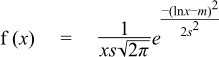#Boost C++ Libraries

...one of the most highly regarded and expertly designed C++ library projects in the world.

#### Log Normal Distribution

`#include <boost/math/distributions/lognormal.hpp>`
```namespace boost{ namespace math{

template <class RealType = double,
class Policy   = policies::policy<> >
class lognormal_distribution;

typedef lognormal_distribution<> lognormal;

template <class RealType, class Policy>
class lognormal_distribution
{
public:
typedef RealType value_type;
typedef Policy   policy_type;
// Construct:
lognormal_distribution(RealType location = 0, RealType scale = 1);
// Accessors:
RealType location()const;
RealType scale()const;
};

}} // namespaces
```

The lognormal distribution is the distribution that arises when the logarithm of the random variable is normally distributed. A lognormal distribution results when the variable is the product of a large number of independent, identically-distributed variables.

For location and scale parameters m and s it is defined by the probability density function:The location and scale parameters are equivalent to the mean and standard deviation of the logarithm of the random variable.

The following graph illustrates the effect of the location parameter on the PDF, note that the range of the random variable remains [0,+∞] irrespective of the value of the location parameter:The next graph illustrates the effect of the scale parameter on the PDF:##### Member Functions
```lognormal_distribution(RealType location = 0, RealType scale = 1);
```

Constructs a lognormal distribution with location location and scale scale.

The location parameter is the same as the mean of the logarithm of the random variate.

The scale parameter is the same as the standard deviation of the logarithm of the random variate.

Requires that the scale parameter is greater than zero, otherwise calls domain_error.

```RealType location()const;
```

Returns the location parameter of this distribution.

```RealType scale()const;
```

Returns the scale parameter of this distribution.

##### Non-member Accessors

All the usual non-member accessor functions that are generic to all distributions are supported: Cumulative Distribution Function, Probability Density Function, Quantile, Hazard Function, Cumulative Hazard Function, mean, median, mode, variance, standard deviation, skewness, kurtosis, kurtosis_excess, range and support.

The domain of the random variable is [0,+∞].

##### Accuracy

The lognormal distribution is implemented in terms of the standard library log and exp functions, plus the error function, and as such should have very low error rates.

##### Implementation

In the following table m is the location parameter of the distribution, s is its scale parameter, x is the random variate, p is the probability and q = 1-p.

Function

Implementation Notes

pdf

Using the relation: pdf = e-(ln(x) - m)2 / 2s2 / (x * s * sqrt(2pi))

cdf

Using the relation: p = cdf(normal_distribtion<RealType>(m, s), log(x))

cdf complement

Using the relation: q = cdf(complement(normal_distribtion<RealType>(m, s), log(x)))

quantile

Using the relation: x = exp(quantile(normal_distribtion<RealType>(m, s), p))

quantile from the complement

Using the relation: x = exp(quantile(complement(normal_distribtion<RealType>(m, s), q)))

mean

em + s2 / 2

variance

(es2 - 1) * e2m + s2

mode

em + s2

skewness

sqrt(es2 - 1) * (2 + es2 )

kurtosis

e4s2 + 2e3s2 + 3e2s2 - 3

kurtosis excess

e4s2 + 2e3s2 + 3e2s2 - 6

 Copyright © 2006-2010, 2012, 2013 Paul A. Bristow, Christopher Kormanyos, Hubert Holin, Bruno Lalande, John Maddock, Johan Råde, Gautam Sewani, Benjamin Sobotta, Thijs van den Berg, Daryle Walker and Xiaogang Zhang Distributed under the Boost Software License, Version 1.0. (See accompanying file LICENSE_1_0.txt or copy at http://www.boost.org/LICENSE_1_0.txt)